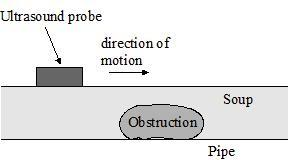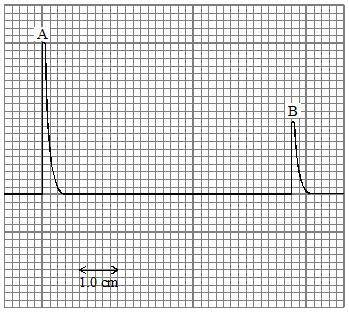# Ultrasound Calculation

## Homework Statement

A food packaging factory is moving soup through a $0.075 m$ diameter pipe when an obstruction occurs in the pipe. An ultrasound probe, connected to an oscilloscope, is moved along the pipe to find the obstruction (Figure 1). The oscilloscope trace is shown below
(Figure 2).

Figure 1:Figure 2:Oscilloscope time base = $20 \times 10^{-6} s \ cm^{-1}$. On figure 2, pulse A is the outgoing signal from the probe and pulse B is the reflected signal from the other side of the pipe, Calculate the speed of the ultrasound in the liquid in the pipe.

## Homework Equations

$v = \frac{s}{t}$

## The Attempt at a Solution

I understand which formula to use but I am struggling to obtain values from the graph shown. The wording has confused me. I would have thought that the time is $(6) \times (20 \times 10^{-6})$ but it is incorrect.

The correct calculation is:
$v = \frac{s}{t}$
$= (150 \times 10^{-3} \ \mathrm{(m)}) \div (132 \times 10^{-6} \ \mathrm{(s)})$

How did the mark scheme obtain the value for the calculations? Thanks in advance.

Hootenanny
Staff Emeritus
Gold Member
What is the total distance travelled by the pulse?

How long does it take the pulse to travel this distance?

How do I calculate total distance? Is the gap between the peaks? Or, is that the time?

Hootenanny
Staff Emeritus
Gold Member
How do I calculate total distance?
The question can only be answered assuming that the obstruction is small compared with with pipe. In other words, assume that the pulse travels from the emitter to the other side of the pipe and back again.
Is the gap between the peaks? Or, is that the time?
The gap between the peaks is indeed the time taken for the pulse to traverse the distance.

Diameter $=0.075m$ hence for the ultraviolet to travel there and back would mean a distance of $0.075m \times 2 = (150 \times 10^{-3}(m))$.

There is an oscilloscope time base of $20 \times 10^{-6} s \ cm^{-1}$. What is that?

Hootenanny
Staff Emeritus
Gold Member
Diameter $=0.075m$ hence for the ultraviolet to travel there and back would mean a distance of $0.075m \times 2 = (150 \times 10^{-3}(m))$.
CorrectThere is an oscilloscope time base of $20 \times 10^{-6} s \ cm^{-1}$. What is that?
That means that every cm on the oscilloscope screen represents 20x10-6 seconds. If your unsure of what a quantity means, it's always a good idea to check the units: s/cmAlso, when calculating the time, do we count from the start of one peak to the start of the other peak? Or, do we start from end of first peak to the start of second peak?

Hootenanny
Staff Emeritus
Gold Member
Also, when calculating the time, do we count from the start of one peak to the start of the other peak? Or, do we start from end of first peak to the start of second peak?
It doesn't matter where you measure from provided that you measure between the equivalent points on each peak.

Ok, then the time is $6.6 \times (20 \times 10^{-6}) = 132 \times 10^{-6}$.

Brilliant, Thanks Hootenanny, I got the values, that the mark scheme has written so the calculation:

$v = \frac{s}{t}$
$= (150 \times 10^{-3} \ \mathrm{(m)}) \div (132 \times 10^{-6} \ \mathrm{(s)})$
$= 1140 ms^{-1}$

Hootenanny
Staff Emeritus
Ok, then the time is $6.6 \times (20 \times 10^{-6}) = 132 \times 10^{-6}$.
$v = \frac{s}{t}$
$= (150 \times 10^{-3} \ \mathrm{(m)}) \div (132 \times 10^{-6} \ \mathrm{(s)})$
$= 1140 ms^{-1}$